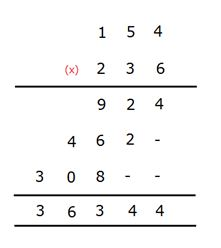# SBI PO Reasoning Questions 2019 (Day-20) High Level-New Pattern

SBI PO 2019 Notification is about to come and it is the most awaited exam among the aspirants. We all know that new pattern questions are introducing every year in the SBI PO exam. Further, the questions are getting tougher and beyond the level of the candidate’s expectations.

Our IBPS Guide is providing High-Level New Pattern Reasoning Ability Questions for SBI PO 2019 so the aspirants can practice it on a daily basis. These questions are framed by our skilled experts after understanding your needs thoroughly. Aspirants can practice these high-level questions daily to familiarize with the exact exam pattern. We wish that your rigorous preparation leads you to a successful target of becoming SBI PO.

#### “Be not afraid of growing slowly; be afraid only of standing still”

[WpProQuiz 5206]

Check here for Video Solutions (Q. 1-5)

Check here for Video Solutions (Q. 6-10)

### Click Here for SBI PO Pre 2019 High-Quality Mocks Exactly on SBI Standard

Directions (1-5): Study the following information carefully and answer the below questions.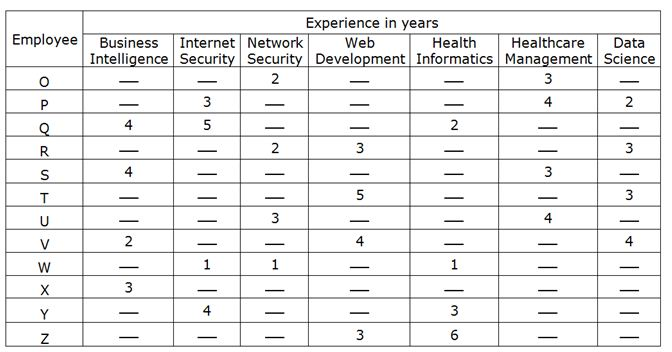Four Teams – Team Red, Team Blue, Team Green and Team Orange – of three members each, have to be created such that they satisfy the following conditions:

S and U have to be together.

A Team cannot have all three members having a common domain of experience.

Team Red includes W and Y.

The total “Web development” experience in Team Blue is 8 years.

The total experience of one team is 27, which is highest among all teams and Team Orange has total experience of 17 years, which is least among all teams. The other two teams have different total years of experience.

None of the members in Team Green have any experience in Data Science.

If P is in a team, then U cannot be in the same team.

1) What is the total experience of all employees in Healthcare management of Team Orange?

a) 10 years

b) 5 years

c) 8 years

d) 7 years

e) None of these

2) What is the total experience of all employees in Team Green?

a) 19 years

b) 23 years

c) 20 years

d) 27 years

e) None of these

3) Who among the following does not belongs to Team Blue?

a) T

b) Q

c) Z

d) R

e) Both Z and Q

4) If P is related to Z in a certain way; and X is related to W in the same way. Then who among the following is T related to?

a) Q

b) R

c) V

d) W

e) Z

5) Which among the following team has people with maximum experience in Internet security?

a) Team Orange

b) Team Blue

c) Team Red

d) Both Team Blue and Team Red

e) Both Team Green and Team Red

(Directions 6–10): Study the following information carefully and answer the questions given below it.

A student from a central university develops a scientific calculator for specific encrypted calculation. Digits from 0 to 9 are coded as different letters, and perform a 3×3 multiplication as shown below in the figure.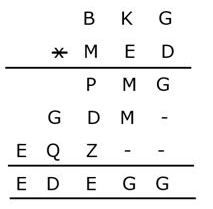Note: No two digits are coded as with same letter.

Carefully observe above calculation and answer the following question based on same multiplication.

6) What is the possible value of “P2 + 2M”?

a) 55

b) 76

c) 65

d) 52

e) None of these

7) What is the possible value of “D3 + 4Q – 2Z”?

a) 226

b) 312

c) 117

d) 200

e) None of these

8) If “GKB” is multiplied by “DEM” then what is the possible resultant of the multiplication?

a) EGBPBM

b) MZKQEM

c) GMPKQZ

d) GEPMBM

e) Can’t be determined

9) What is the possible value of “4P + 3G – 3B”?

a) 27

b) 28

c) 45

d) 34

e) None of these

10) If “PZ” is multiplied by “BQ” then what is the possible resultant of the multiplication?

a) MKED

b) QPZQ

c) DGQD

d) EKEG

e) Can’t be determined

Directions (1-5):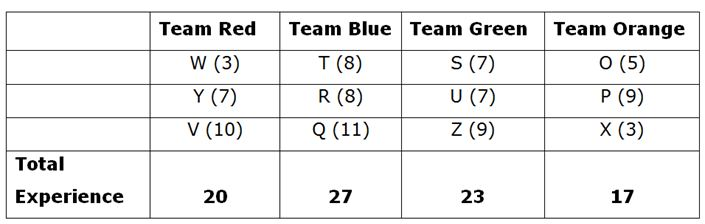From the given details in the table we can conclude the following;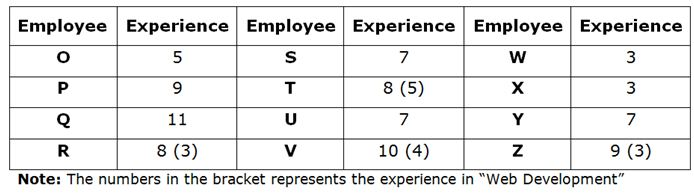A Team cannot have all three members having a common domain of experience.

• Team Red includes W and Y.
• The total “Web development” experience in Team Blue is 8 years.

So, it must be (T + R/Z) = 8;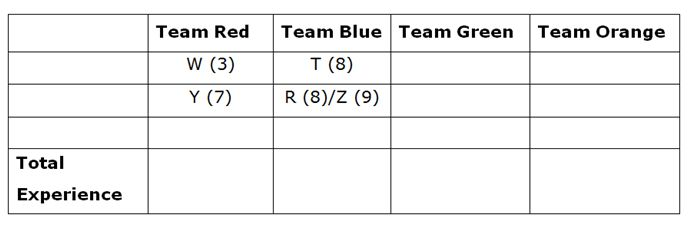• The total experience of one team is 27, which is highest among all teams and Team Orange has total experience of 17 years, which is least among all teams. The other two teams have different total years of experience.
• S and U have to be together.
• None of the members in Team Green have any experience in Data Science.
• If P is in a team, then U cannot be in the same team.

The possible combination to get 27 years of experience is 11+9+7(this is not possible as S and U should be together), 8+8+11 and 10+8+9.

The possible combination to get 17 years of experience is 5+9+3 and 7+7+3.

We get the following cases. Also in Case (2) T, Z and V has a common domain experience this case gets eliminated.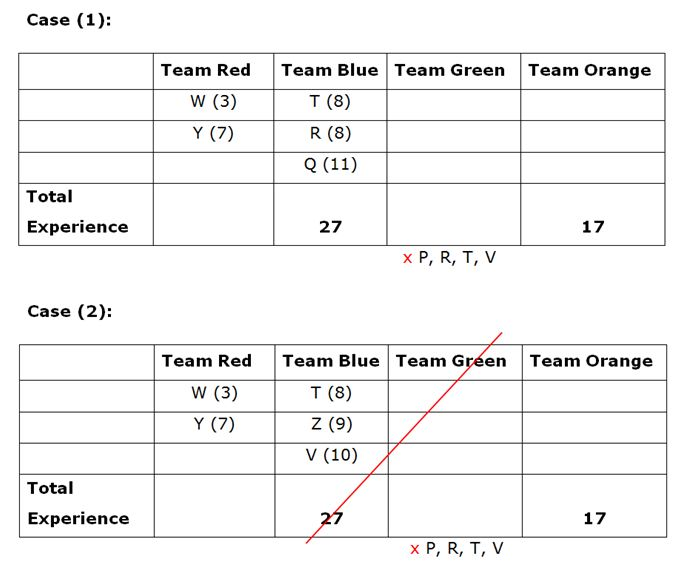Since, the possible combination to get 17 years of experience is 5+9+3 and 7+7+3 also S and U have to be together.

So we get two possibilities Case (1a) and Case (1b),

In Case (1a), we cannot fix the employee Z (9) at Team Orange as the remaining P and V cannot be at Team Green.

Case (1b) gets eliminated as either P or V should be in Team Green which doesn’t satisfy the statements. So, this case gets eliminated.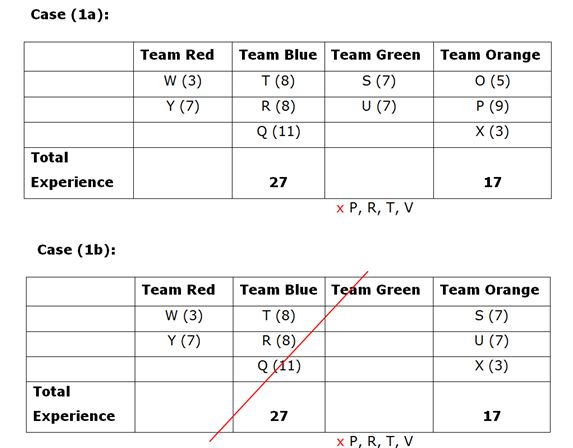Hence, the final arrangement is,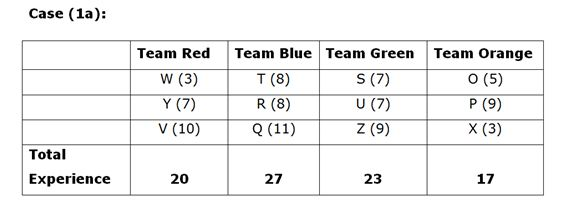4) Answer: b) (All the pair has same year of experience)

Directions (6-10):

We have: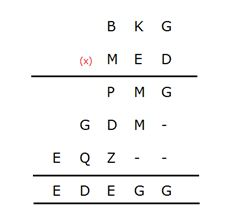From the above multiplication process; we can conclude the following: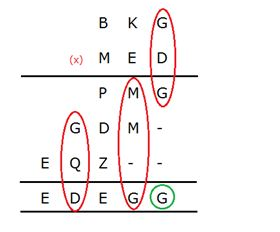• G x D = G, G + Q = D and M + M = G;

Since M + M = G; also there is no carry in addition (mentioned in green), we can conclude that G must be an even number.

So, the possibilities were,

If, (G=2/4/6/8)

M should be (1+1/2+2/3+3/4+4)

Since, G x D = G; the even digit (G) multiplied by a digit (D) should give the same digit (G).

So the possibilities were,

2 x 6 = 12 à Case (1)

4 x 6 = 24 à Case (2)

8 x 6 = 48 à Case (3)

From all the cases we can conclude that D=6.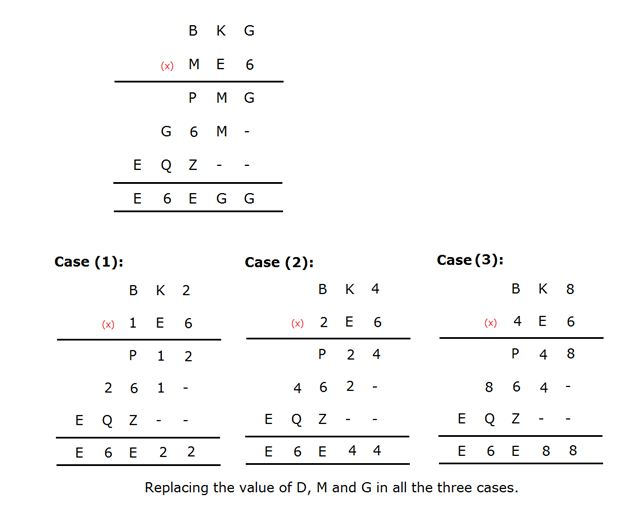• Since there is no carry in the last digit (marked in red); we can conclude that G+Q=D (marked in green).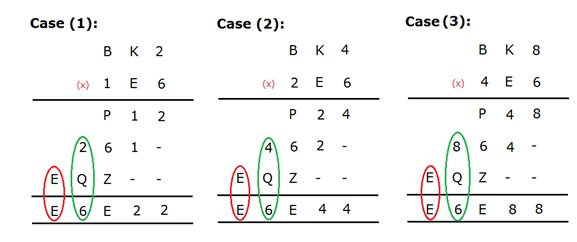So,

In Case (1): 2 + (4/3) = 6 (there can be carry digit added); 1 is omitted as the value of M =1.

In Case (2): 4 + (1/0) = 6 (there can be carry digit added); 2 is omitted as the value of M =2.

In Case (3): There is no such possibility so it can be eliminated.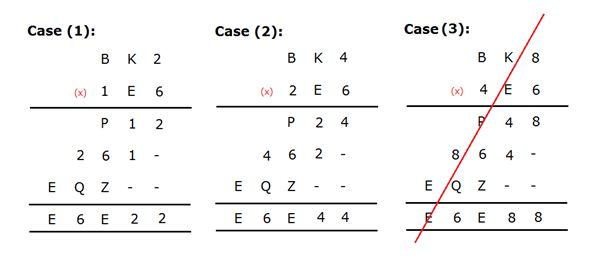In Case (1): Since the value of (2 x E = 1) is not possible it gets eliminated (marked in green).

In Case (2): The value of (4 x E = 2); we get two possibilities.

(4 x 3 = 12) –> Case (2a)

(4 x 8 = 32) –> Case (2b)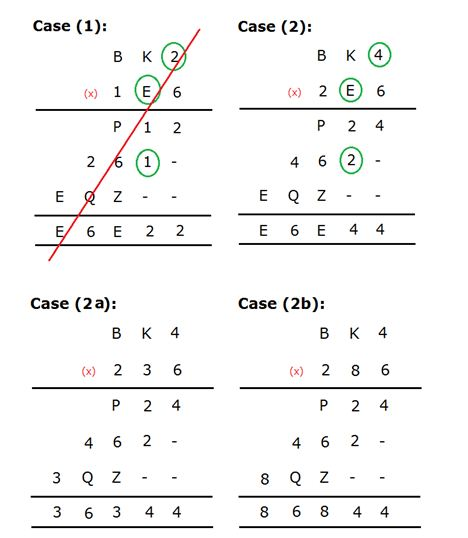In Case (2a): 3 x 4 = 12; then (K x 3)+1 = 6. There is no possibility other than substituting 5 as the value of K. So, we can conclude the value of K as 5.

In Case (2b): 8 x 4 = 32; then (K x 8)+3 = 6. There is no possibility of getting odd digit in multiple of 8. So, it gets eliminated.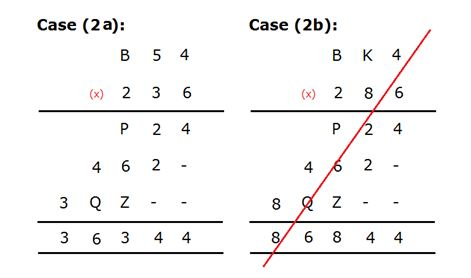In Case (2a), we get (B54 x 3 = 462). So we can conclude the value of B as 1.

So, the process becomes,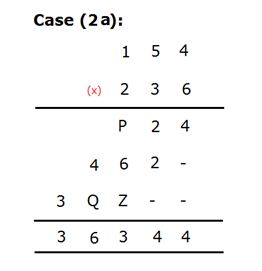So, by multiplying 154 x 236; we can find the value of P, Q and Z.

The final solution is,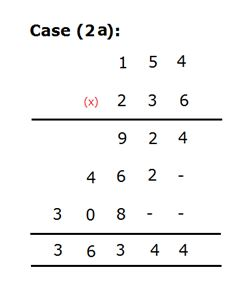We have:

Clearly, P = 8 & M = 2.

Thus, value of “P2 + 2M” = (9×9 + 2×2) = 85.

Hence, option E is correct choice.

Clearly, D = 6, Q = 0 and Z = 8.

Thus, value of “D3 + 4Q – 2Z” =(63 + 4×0 – 2×8) = 496.

Hence, option D is correct choice.

We have:

B = 1, K = 5, G = 4, D =6, E = 3, M = 2, P = 9, Z = 8, Q = 0.

Thus, “GKB” = 451 & “DEM” = 632

Thus, after multiplication of “451” with “632” we get:

(451 x 632) = 285032

Thus, “285032” is coded as “MZKQEM”.

Hence, option B is correct choice.

We have:

P = 9, G = 4 & B = 1.

Thus, value of “4P + 3G – 3B” = (9×4 + 3×4 -3×1) = 45.

Hence, option C is correct choice.

We have:

B = 1, P = 9, Z = 8 and Q = 0.

Thus, “PZ” = 98 & “BQ” = 10

Thus, after multiplication of “98” with “10” we get:

(98 x 10) = 980

Thus, “980” is coded as “PZQ”.

Hence, option B is correct choice.